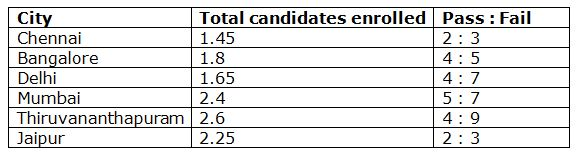# SBI Clerk Prelims 2020 Quantitative Aptitude Questions (Day-09)

Dear Aspirants, Our IBPS Guide team is providing new series of Quantitative Aptitude Questions for SBI Clerk Prelims 2020 so the aspirants can practice it on a daily basis. These questions are framed by our skilled experts after understanding your needs thoroughly. Aspirants can practice these new series questions daily to familiarize with the exact exam pattern and make your preparation effective.

[WpProQuiz 7631]

Ensure Your Ability Before the Exam – Take SBI Clerk 2020 Prelims Free Mock Test

Data Interpretation

Directions (Q. 1 – 5): Study the following information carefully and answer the given questions?

Following table shows the total number of candidates (In lakhs) enrolled for the NEET examination from 6 different cities and also shows the ratio of passed and failed candidates among them.1) Find the total number of pass percentage in Chennai?

a) 40 %

b) 25 %

c) 50 %

d) 35 %

e) None of these

2) Find the total number of failed candidates in Bangalore, Delhi and Jaipur together?

a) 284000

b) 256000

c) 320000

d) 340000

e) None of these

3) Find the difference between the total number of passed candidates from Mumbai and Thiruvananthapuram together to that of total number of candidates enrolled from Chennai?

a) 48000

b) 35000

c) 52000

d) 26000

e) None of these

4) If the ratio of male to that of female candidates enrolled for the examination from Chennai is 3 : 2, then find the ratio between the total number of male candidates enrolled for the examination from Chennai to that of total number of candidates enrolled for the examination from Bangalore?

a) 29 : 60

b) 35 : 72

c) 44 : 91

d) 57 : 113

e) None of these

5) Total number of candidates enrolled for the examination from Delhi and Mumbai together is approximately what percentage of total number of candidates enrolled for the examination from Thiruvananthapuram and Jaipur together?

a) 70 %

b) 50 %

c) 85 %

d) 100 %

e) 115 %

Approximation

Directions (Q. 6 – 10): What approximate value should come in place of question mark in the following questions?

6) 79.989 % of 599.99 + 32.99 % of 400.009 – 48.065 % of 149.98 = ?

a) 580

b) 520

c) 540

d) 560

e) 640

7) √4760.998 × 2.995 + 49.98 % of 25.909 + 15.086 × 1.9998 = ?

a) 220

b) 250

c) 280

d) 310

e) 420

8) ? + (6.02)3 – 29.03 * 7.980 = 1.998 * 1.998

a) 30

b) 20

c) 40

d) 10

e) 50

9) 13.78 % of 3048 – (5/9.21) of (7/2.5) of 71 = 23.2 + ?

a) 356

b) 378

c) 414

d) 292

e) 480

10) √287 × 14 ÷ 6.92 = ?2 + (8.13)1/3 + 27.89 ÷ 4

a) 9

b) 7

c) 5

d) 11

e) 13

Directions (1-5):

Total candidates enrolled = 145000

Total number of passing candidates = 145000*(2/5) = 58000

Required % = (58000/145000)*100 = 40 %

(Or)

The ratio of passed to that of failed candidates = 2 : 3 (2x, 3x)

Required % = (2x/5x)*100 = 40 %

The total number of failed candidates in Bangalore, Delhi and Jaipur together

= > 180000*(5/9) + 165000*(7/11) + 225000*(3/5)

= > 100000 + 105000 + 135000 = 340000

The total number of passed candidates from Mumbai and Thiruvananthapuram together

= > 240000 *(5/12) + 260000*(4/13)

= > 100000 + 80000 = 180000

The total number of candidates enrolled from Chennai = 145000

Required difference = 180000 – 145000 = 35000

The ratio of male to that of female candidates enrolled for the examination from Chennai = 3 : 2 (3x, 2x)

The total number of male candidates enrolled for the examination from Chennai

= > 145000*(3/5) = 87000

The total number of candidates enrolled for the examination from Bangalore

= > 180000

Required ratio = 87000 : 180000 = 29 : 60

Total number of candidates enrolled for the examination from Delhi and Mumbai together

= > 1.65 + 2.4 = 4.05 lakhs = 405000

Total number of candidates enrolled for the examination from Thiruvananthapuram and Jaipur together

= > 2.6 + 2.25 = 4.85 lakhs = 485000

Required % = (405000/485000)*100 = 83.5 % = 85 %

Directions (6-10):

79.989 % of 599.99 + 32.99 % of 400.009 – 48.065 % of 149.98 = ?

80/100 * 600 +33/100 * 400 – 48/100 * 150 =?

480+132-72=?

540=?

√4760.998 × 2.995 + 49.98 % of 25.909 + 15.086 × 1.9998 = ?

√4761 * 3 + 50/100 * 25 + 15 * 2=?

69*3 + 13 + 30 = ?

207 + 43 = ?

250 = ?

? + (6.02)3 – 29.03 * 7.980 = 1.998 * 1.998

? + 216 – 29 * 8 = 2 * 2

? + 216 – 232 = 4

? = 4 + 232 – 216

? = 20

14 % of 3050 – (5/9) of (7/2.5) of 72 = 23 + ?

(14/100) * 3050 – (5/9) * (7/2.5) * 72 = 23 + ?

427 – 112 – 23 = ?

? = 292

√289 × 14 ÷ 7 = ?2 + (8)1/3 + 28 ÷ 4

17 * (14/7) = ?2 + 2 + (28/4)

34 – 2 – 7 = ?2

?2 = 25

? = 5

 Check Here to View SBI Clerk Prelims 2020 Quantitative Aptitude Questions Day 08 Day 07 Day 06 Click Here for SBI Clerk 2020 – Detailed Exam Notification